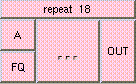REPEATTHE BOX'S MAIN HELP: This is function returns mod(A*FQ, 1). This is the percentage of 1, which that frequency of A represents. A (input): The varying value to be divided up by FQ. This must be a float. FQ (input): This must be a float. This is the frequency value. OUT (output): This is function returns mod(A*FQ, 1). This is the percentage of 1, which that frequency of A represents.

<-- BACK TO Math